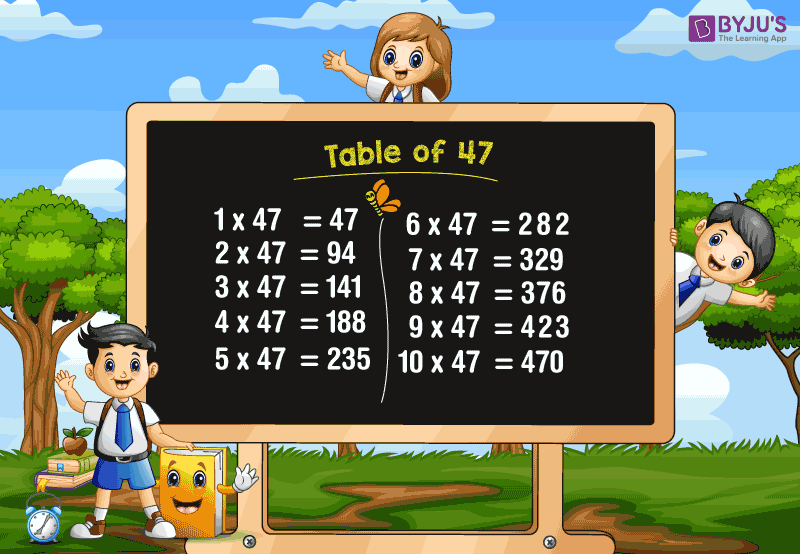# Table of 47

The Table of 47 or a maths multiplication table of forty-seven (47) is given here along with chart and PDF. Students can access this table to solve the multiplication and division problems easily. Get all the Tables from 1 to 100 here. Memorization of tables helps in time saving while attempting the time-based exams.

### Download Table of 47 HereWhat is the table of 47?

The table of 47 is the multiplication of 47 with counting numbers and it can also be written using repeated addition of the number itself, i.e. 47.

Chiku wants to distribute candies to his 47 classmates. If he decided to give 5 each, then how many candies would he need?

Total number of candies must be 47 × 5.

i.e. 47 + 47 + 47 + 47 + 47 = 235

Therefore, 47 × 5 = 235

Similarly, one can calculate all the multiples of 47. Let’s have a look at the table of multiplication of forty-seven from 1 to 20 given below.

## Multiplication Table of 47

 47 × 1 = 47 47 × 2 = 94 47 × 3 = 141 47 × 4 = 188 47 × 5 = 235 47 × 6 = 282 47 × 7 = 329 47 × 8 = 376 47 × 9 = 423 47 × 10 = 470 47 × 11 = 517 47 × 12 = 564 47 × 13 = 611 47 × 14 = 658 47 × 15 = 705 47 × 16 = 752 47 × 17 = 799 47 × 18 = 846 47 × 19 = 893 47 × 20 = 940

### How to Read the Table of 47

Read the below forty-seven (47) times table from 1 to 10.

• One time forty-seven is forty seven
• Two times forty-seven is ninety four
• Three times forty-seven is one hundred and forty one
• Four times forty-seven is one hundred and eighty eight
• Five times forty-seven is two hundred and thirty five
• Six times forty-seven is two hundred and eighty two
• Seven times forty-seven is three hundred and twenty nine
• Eight times forty-seven is three hundred and seventy six
• Nine times forty-seven is four hundred and twenty three
• Ten times forty-seven is four hundred and seventy

### Get More Multiplication Tables

Keep visiting www.byjus.com and download BYJU’S – The Learning App to learn maths concepts in an easy and engaging way.